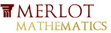# Effect of Initial Value on Graph of Exponential Function (C>0)This material was originally developed through Merlot
as part of its collaboration with the SERC Pedagogic Service.

## Summary

This classroom activity presents College Algebra students with a ConcepTest and a Question of the Day activity concerning the effect of the initial value, C, on the y-intercept and position of an exponential graph where C>0 and k is an arbitrarily fixed value in f(x)=Ce^(kx).

While students may have learned that the initial value affects the shape of a exponential curve, most do not realize exactly how or that it also affects the position of the y-intercept. Analyzing the effects requires some effort on the students' part but the results are rewarding.

## Learning Goals

To enable students to:

• develop their understanding of the effects of the initial value in an exponential function
• exercise their mathematical intuition and verify it via appropriate calculations
• exercise the skills of algebraic and mathematical analysis

## Context for Use

This activity can be carried out in either a small College Algebra class or a large lecture setting anytime during or after the topic of graphing exponential functions has been covered.

The activity is comprised of three segments: ConcepTest, Question of the Day and Conclusion, involving the use of a demonstration applet. The time required for the entire activity is approximately 25 minutes but fewer segments can be offered as a shorter alternative (see Activity Description below for individual segment times).

## Description and Teaching Materials

Activity Description and Teaching Materials

• Instructor presents a ConcepTest (Rich Text File 73kB Aug18 10) in the form of a straw poll (either show-of-hands or written) concerning the effect of increasing the initial value, C, on the position of the y-intercept and the position of the graph with regard to quadrants. Each student is asked to make a conjecture and the instructor records the results for the class to see. (~5 minutes)
• After the straw poll, the question becomes the Question of the Day (Rich Text File 70kB Aug18 10) and students work in pairs to share and explain their reasoning in written form. (~10 minutes)
• In conclusion, the instructor presents a classroom demo involving a Java applet that quickly and easily demonstrates the effects of changing the initial value in an exponential function.
http://www.ltcconline.net/greenl/java/Other/MovingGraph/MovingGraph.html
In addition, the instructor ensures that students understand the algebraic and mathematical analysis involved and clearly explains the reasons for the effects. (~10 minutes)

## Teaching Notes and Tips

Student understanding of the role of initial value and proportionality constant in exponential functions is limited at best. Most have not considered the y-intercept in terms of the values of C and k, and many have difficulty in analyzing the effects of changing the values of these terms. The fact that using a negative value for k reflects the graph around the y-axis seems to escape them. This activity is designed to help them develop a better grasp of the nature of exponential functions.

## Assessment

I generally give the students a grade for participation in this activity and sometimes grade their final calculations concerning the work required.

## References and Resources

Larry Green's Applet Page-Parameters and Graphs
This applet allows the user to change the initial value and the proportionality constant of an exponential function and immediately see the graphical results.
http://www.ltcconline.net/greenl/java/index.html
http://www.ltcconline.net/greenl/java/Other/MovingGraph/MovingGraph.html
MERLOT description of this resource
http://www.merlot.org/merlot/viewMaterial.htm?id=77279